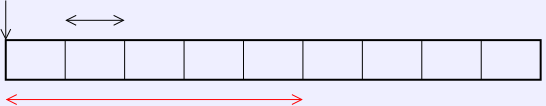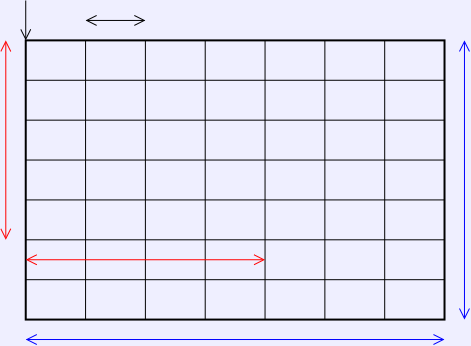Array Location ArithmeticArrays are typically layed out continguously in memory. The basic parameters are:
 α The starting address of the array. e The size of an array element. lb, ub The lower and upper array bounds. a[i] The subscript expression being evaluated.

So, for a one-dimensional array,α
a[i]
a[lb..ub]
e
i-lb slots

The address of address of the location a[i] is given by:

addr(a[i]) = α + e(i − lb) = (α − elb) + ei

This can be generalized for a two-dimensional array. Note that the first cell of each row is located in memory just after the last cell of the row above it.α
a[i,j]
e
a[lb1..ub1lb2..ub2]
i−lb1
rows
j−lb2 slots
ub1lb1 + 1 rows
ub2lb2 + 1 columns

So the address of a[i] will be given by starting from α and first skipping the rows above it, then the cells to its left.

addr(a[i,j]) = α + e(ub2lb2+1)(ilb1) + e(jlb2)
= (α − eub2lb1 + elb2lb1 − elb1 − elb2) + i(eub2 − elb2 + e) + ej

Languages which create multi-dimensional arrays as shown here typically require that the bound be constants. That means that the address formulas can be reduced at compile to time to a linear computation of the subscripts (the sum of a constant and a constant multiple of each subscript).

In languages like C and Java, where the lower bound is zero, and the upper bound is the size less 1, we can substitute 0 for each lb and s−1 for each ub and get:

addr(a[i]) = α + ei
addr(a[i,j]) = α + s2ei + ej